Matlab Source Code for Species Transport through Nafion Membranes in Direct Ethanol, Direct Methanol, and Direct Glucose Fuel Cells | OMICS International
Journal of Physical Mathematics

# Matlab Source Code for Species Transport through Nafion Membranes in Direct Ethanol, Direct Methanol, and Direct Glucose Fuel Cells

Summerfield JH* and Manley MW

Department of Chemical and Physical Sciences, Missouri Southern State University, USA

*Corresponding Author:
Summerfield JH
Department of Chemical and Physical Sciences
Missouri Southern State University, USA
Tel: 417-625-9717
E-mail: [email protected]

Received Date: November 14, 2016; Accepted Date: November 28, 2016; Published Date: November 30, 2016

Citation: Summerfield JH, Manley MW (2016) Matlab Source Code for Species Transport through Nafion Membranes in Direct Ethanol, Direct Methanol, and Direct Glucose Fuel Cells. J Phys Math 7:203. doi: 10.4172/2090-0902.1000203

Copyright: © 2016 Summerfield JH, et al. This is an open-access article distributed under the terms of the Creative Commons Attribution License, which permits unrestricted use, distribution, and reproduction in any medium, provided the original author and source are credited.

Visit for more related articles at Journal of Physical Mathematics

#### Abstract

A simple simulation of chemical species movement is presented. The species traverse a Nafion membrane in a fuel cell. Three cells are examined: direct methanol, direct ethanol, and direct glucose. The species are tracked using excess proton concentration, electric field strength, and voltage. The Matlab computer code is provided.

#### Keywords

Mat lab; Fuel cells; Polymers

#### Introduction

An efficient fuel cell could replace combustion engines and advance the goal of being less oil dependent. For a fuel cell, an accurate mathematical model is an important tool for optimizing fuel cell efficiency, which makes fuel cell model a current research topic [1,2]. The novelty of this work is that three fuel cells are modelled apart from the typical hydrogen oxygen fuel cell. Furthermore, a Matlab computer program is presented so that others may investigate their own systems.

The systems studied in this work involve chemical species transport with an ion exchange polymer composite matrix supported on an electrode surface. The ion exchange polymer that is used to modify electrodes in this work is Nafion, the structure of which is shown in Figure 1.

Structurally Nafion is a Teflon-like, hydrophobic, fluorocarbon backbone with sidechains that terminate in a hydrophilic sulfonic acid. When Nafion is in contact with solution, the proton from the sulfonic acid can easily exchange with cationic species in solution. Nafion provides a cation selective matrix where cationic redox species are concentrated within the film. Because Nafion is a polymer, mass transport in the system is slowed. In the acid form, Nafion provides ion conduction through the acidic proton about the sulfonic acid.

A fuel cell is similar to a battery in that it converts chemical energy into electrical energy and is better than a battery because it does not undergo charge/discharge cycles. A fuel cell provides power as long as it is provided fuel, similar to a combustion engine. A fuel cell is better than a combustion engine because it converts chemical energy directly into electrical energy without moving through a pressurevolume cycle and so is a more efficient process. The Carnot limitations restrict combustion engines to a theoretical maximum efficiency of 40%. Because a fuel cell converts chemical energy to electrical energy without mechanical cycles, there are no thermodynamic limitations and so the theoretical efficiency is 100%.

A fuel cell consists of two electrodes separated by an ion conducting membrane. As is typical, the membrane is Nafion and the electrodes are graphite. When catalyst coated electrodes are pressed against the membrane, interfacial zones are created. The electrochemical reactions occur only in these interfacial zones. A local difference in the concentration of anions and cations is produced because of these reactions. This separation of charge creates a potential difference across the cell. The slightly resistive nature of the electrodes and Nafion causes a potential loss in these regions. Table 1 shows the fuel cells investigated in this work .

Fuel Cell Type Anode Cathode Voltage (V)
Methanol CH3OH + 6OH–⇒ CO2 + 5H2O + 6e 3/2O2 + 3H2O + 6e⇒ 6OH– 1.12
Ethanol CH3CH2OH + 2OH–⇒ CH3COOH + 3H2O + 4e,
CH3CH2OH + 12OH–⇒ 2CO2 + 9H2O + 12e
3O2 + 6H22 + 12e⇒ 12OH– 1.17
Glucose C6H12O6+ H2O⇒ C6H12O7 + 2H++ 2e O2+ 2H++ 2e⇒ H2O 1.30

Table 1: The fuel cell type, the anode reaction, the cathode reaction, and the cell voltages for this work.

#### The Model

Consider a one dimensional model. Define Cj(x, t) as the concentration in mol/cm3, Jj(x, t) as the flux (mol cm–2 s–1) and φ(x, t) as the potential (volts). The Nernst-Plank equation relates these quantities.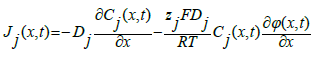(1)

Where Dj is the diffusion rate of species j (cm2 s–1), zj is the charge on species j, F is Faraday’s constant (C mol–1), R is the gas law constant (J K–1 mol–1), and T is temperature (K).

More specifically, Cj(x, t) is the concentration of j needed to neutralize the sulfonates concentration. Let N be the concentration of sulfonates in the Nafion.

For simplification, the relation between flux and concentration is relied on,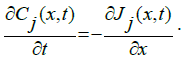(2)

Using Eq. (1), Eq. (2), and our newest definitions,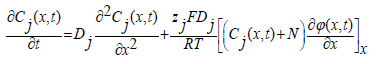(3)

The total current, i, at steady state is set by the steady state flux, Jj,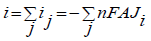(4)

where n is the number of electrons involved in the redox reaction and A is the surface area of the electrode. This work considers each chemical species independently so Eq. (4) reduces to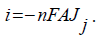(5)

Turning to the relation between concentration and electric potential energy, Poisson’s equation is relied on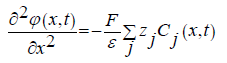(6)

where ε=ε0εr and is the relative permittivity. ε0 is the vacuum permittivity and εr is the dielectric constant. This is 20 for Nafion . For the systems of interest, Eq. (6) becomes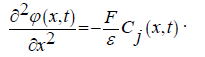(7)

Finally then, equations (3) and (7) are used to define the species movement over the Nafion membrane .

Equation (3) provides the transport equation with a condition on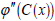as seen in Eq. (7). Instead of considering derivatives of the potential, it is replaced with a relation to the electric field, E(C(x))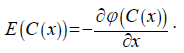(8)

This transforms Eq.(1) to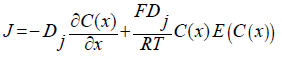(9)

where J is the time independent, steady state. Equation (7) can also be recast,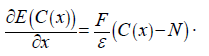(10)

Equations (9) and (10) appear to have no simulation problems. After a bit of examination they reveal themselves to be stiff partial differential equations. Standard methods were used to regularize them  and a Runge-Kutta scheme was used for the simulation’s calculations .

Let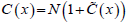then the above become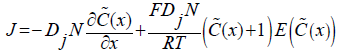(11)

and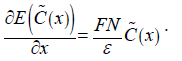(12)

Let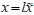where l is the Nafion film thickness in cm and the above can be rewritten as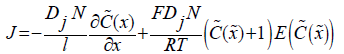(13)

and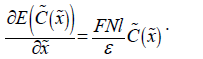(14)

It was thought that the electric field values might be too large and create problems in the simulation so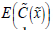was scaled by 10–3. That is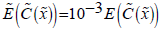. This scaling and rearrangement yields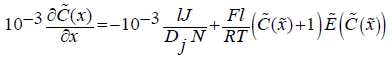(15)

and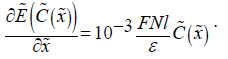(16)

Simplifications can now take place. Equations (15) and (16) now become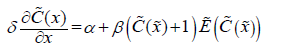(17)

and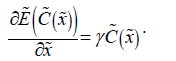(18)

Where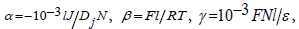and δ=10–3.

The simplifications continue. Let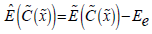where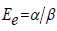. Then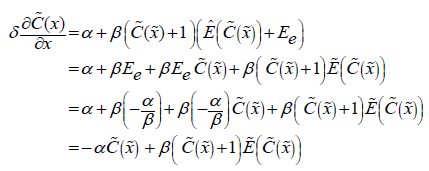(19)

and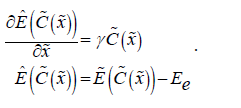(20)

Finally let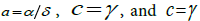along with writing each equation as a function ofand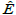.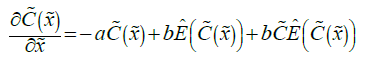(21)

and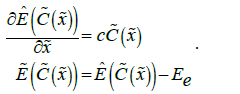(22)

Equations (21) and (22) are the equations used in the Appendix A program. The diffusion coefficients shown in Table 2.

Fuel Cell Type Diffusion Coefficient (cm2/s–1) Reference
Methanol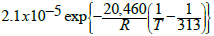Ethanol 1.83 x10−5 
Glucose 6.5 x10−6 

Table 2: Diffusion coefficients for the simulations. R is the ideal gas constant and T is temperature. The other parameters are found in the program.

To verify that the simulation results were accurate, an equation with a fixed point in the center of the interval over which the simulation was ran was needed. The equation used was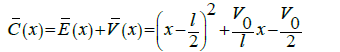(23)

where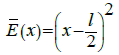and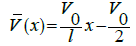(24)

The eigenvalue used in the simulation was the value that resulted from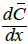evaluated at x = l/2. These equations were entered into the simulation in the form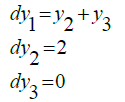(25)

Where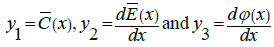. The resulting curves were as expected, creating the correct parabola for y1 and the correct lines for y2 and y3.

#### Results and Discussion

For a 52 micrometer Nafion membrane, the excess proton concentration at the membrane, the electric field strength at the membrane, and the voltage at the membrane are shown for all three fuel cells in Figures 2-10. The simulation currently does not allow a thickness of 51 micrometers or less. This is likely because of the fixed point assumption and for a membrane 51 micrometers or less, one cannot assume the excess proton concentration is zero in the middle of the film or the position of the zero concentration is too uncertain. It is interesting the simulation breaks down at around the same thickness used in a fuel cell. It is not clear if this is the result of the simulation method or a characteristic of the modeling equations or the constraints on the system. Without the fixed point assumption, there is no interval within the film where the concentration of protons and Nafion is neutral. This is why the simulation breaks down and could be why such thin films are not stable in fuel cells.

Figure 2: Excess proton concentration in a 52 micrometer Nafion membrane in a methanol fuel cell.

Figure 3: Electric field strength in a 52 micrometer Nafion membrane in a methanol fuel cell.

Figure 5: Excess proton concentration in a 52 micrometer Nafion membrane in an ethanol fuel cell.

Figure 6: Electric field strength in a 52 micrometer Nafion membrane in an ethanol fuel cell.

Figure 8: Excess proton concentration in a 52 micrometer Nafion membrane in a glucose fuel cell.

Figure 9: Electric field strength in a 52 micrometer Nafion membrane in a glucose fuel cell.

The shapes of the curves across the membrane are similar cell to cell. All are essentially invariant across the membrane. For the excess protons, the membranes show a neutral region across the membrane with steep gradients at boundaries that yield concentration polarizations. That is, protons build up at the anode edge and deplete at the cathode edge shown in Figures 2-10.

#### References

Select your language of interest to view the total content in your interested language

### Article Usage

• Total views: 3333
• [From(publication date):
December-2016 - Jan 18, 2020]
• Breakdown by view type
• HTML page views : 3183Can't read the image? click here to refresh